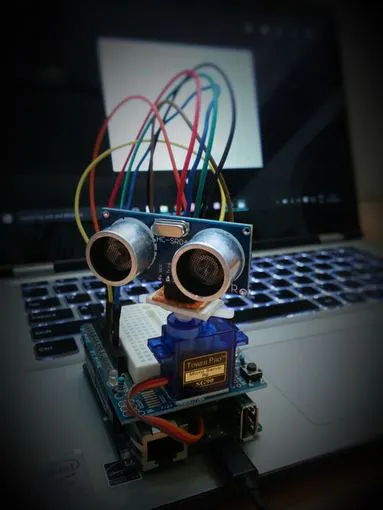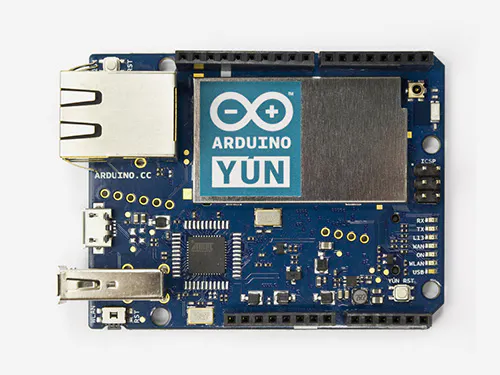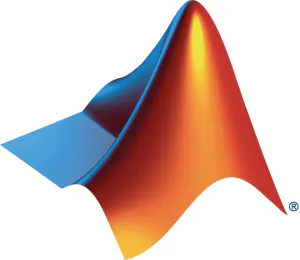# Ultrasonic Map-Maker using an Arduino Yun

Generates maps based on distance between itself and obstacles autonomously and provides visual feedback.

BeginnerFull instructions provided4 hours72,462## Things used in this project

### Hardware componentsArduino Yun
×1
 Ultrasonic Sensor
×1
 Servos (Tower Pro MG996R)
×1Jumper wires (generic)
×10

### Software apps and online servicesArduino IDEMATLAB

## Schematics

### YunConfig.fzz

Fritzing file for servo and Ultrasonic sensor connections

## Code

### matlab_code_to_run_on_pc.m

MATLAB
MatLab code to be compiled and executed on PC
```theta = 0:(pi/180):pi;
s = serial('COM10');
s.BaudRate=9600
fopen(s)
i = 0;

inc = 1;

while i<180
A = fgets(s);
num(i+1) = str2num(A);
i = i+1;
end
fclose(s)

j = 1

while j<181
tab(j,1) = (j-1)*inc
tab(j,2) = num(j)
tab(j,3) = num(j)*cosd((j-1)*inc)
tab(j,4) = num(j)*sind((j-1)*inc)
j = j+1
end
%figure
%polar(theta,num)

plot(tab(:,3),tab(:,4))
```

### Arduino Code

Arduino
Arduino Code to be uploaded to Arduino Yun
```#include <Servo.h>
#include <NewPing.h>

#define TRIGGER_PIN  12
#define ECHO_PIN     11
#define MAX_DISTANCE 200

NewPing sonar(TRIGGER_PIN, ECHO_PIN, MAX_DISTANCE);
Servo myservo;

int pos = 0;
int it = 10;

void setup() {
myservo.attach(9);
Serial.begin(9600);
delay(3000);
}

void loop() {
int i = 0;
int t = 0;
int a = 0;

for (i = 0; i < 180; i ++)
{
unsigned int uS = sonar.ping();
myservo.write(i);
delay(20);
for (t = 0; t < it; t++)
{
uS = sonar.ping();
a = uS/US_ROUNDTRIP_CM + a;
delay(30);
}

a = a / (it-1);
t = 0;

Serial.println(a);
a = 0;
}

}
```

## Credits

### Satyavrat Wagle

6 projects • 49 followers
I have an active interest in IoT, Wireless Sensor Networks, Single Board Computing Implementations, Embedded Systems, and Robotics.